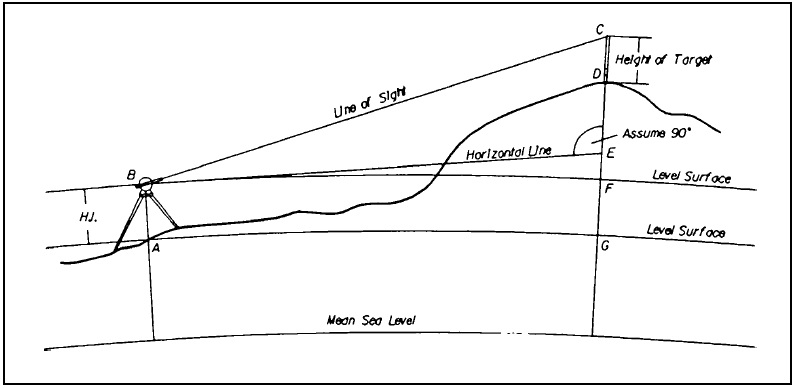# Basic Surveying Theory and Practice - Quiz

## Quiz Question

 1. Which of the following is a type of surveying? Geodetic Surveying Plane Surveying Both a and b None of the above 2. sin 60° = 0.8660 and cos 60° = 0.5 , What is the value of tan 60° ? 1.5321 1.7321 1 1.6321 3. Which of the following is not a Chaining Error? Proportional Errors Constant Errors Wind Correction Temperature Correction 4. What does EDM stand for? Electronic Deviant Measuring Electronic Distance Measuring Electric Distance Monitoring Electro-magnetic Distance Monitoring 5. Repeated measurements of an angle _________ accuracy over that obtained from a single measurement. Decrease Doesn’t affect Increase none of the above 6. A mean zenith angle is obtained by first adding the direct and reverse readings to obtain the algebraic difference between their sum and 360°; then dividing this difference by 2, and algebraically adding the result to the first (direct) series measurement. True False 7. The azimuth of a line on the ground is its __________ angle measured from the meridian to the line. Horizontal Vertical 8. A Traverse is a succession of curved path along or through the area to be surveyed. True False 9. To achieve sub-centimeter accuracies in positions, we need a survey grade receiver and a technique called ________ GPS. Confidential Differential Sub- centimeter Surveyor 10. The effects of Earth Curvature and Atmospheric Refraction must be taken into account when using trigonometric methods to determine elevations. True False 11. Cross sections are used to determine the ________ of the ground surface through the alignment corridor. Shape Size Length None of the above 12. ___________ leveling is the process used to determine a difference in elevation between two points. Defining Determining Differential None of the above 13. A DTM is numerical representation of the configuration of the terrain consisting of a very dense network of points of known X,Y,Z coordinates. What is DTM? Distribution of terrain model Directions in terrain model Digital terrain model Definitive terrain model 14. Spirals are sometimes called _______ curves because they are used to transition into and out of circular curves. Transition Rounded Helical Bi axial 15. The Vertical Offset is calculated by, v = gD2. What is g? g= (G2+G1)/ 2L g= (G2G1)/ 4L g= (G2*G1)/ 4L g= (G2-G1)/ 2L 16. Stationing is usually expressed as number of stations or 100 foot units plus the number of feet less than 100 and any decimal feet. This value is preceded by an alphanumeric alignment designation. A point on an alignment called B3 and 1345.29 feet from the beginning of the stationing would be designated as _______? B3 134+5.29 B3 13+45.29 B3 1345.29 B3 1345.29 17. From the following figure what is EC?EC = AB x cos(zenith angle) EC = BA x sin(zenith angle) EC = BC x cos(zenith angle) EC = BF x cos(zenith angle) 18. A Level is an instrument with a telescope that can be leveled with a ______ bubble. Water Blue Yellow Spirit 19. The Global Positioning System (GPS) is a navigational or positioning system developed by the ____________________? United States Department of Defense United States Department of Transportation United States Department of Roadways United States Department of Technology. 20. The GPS system is a constellation of ______ at an orbit high enough to allow a field of view of several satellites, yet low enough to detect a change in the geometry even if you moved a few feet. Natural stars Manmade stars Earth stars Planets